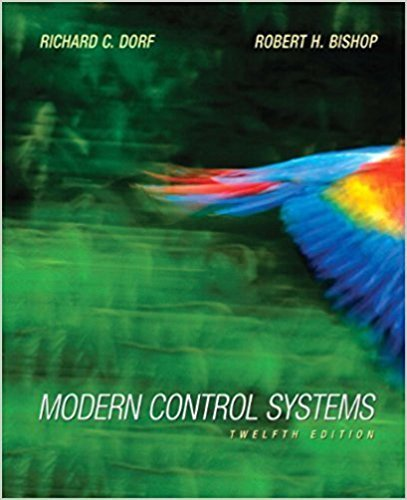×
Get Full Access to Modern Control Systems - 12 Edition - Chapter Chapter 2 - Problem E2.16
Get Full Access to Modern Control Systems - 12 Edition - Chapter Chapter 2 - Problem E2.16

×

# The position control system for a spacecraft platform is governed by the followingISBN: 9780136024583 453

## Solution for problem E2.16 Chapter Chapter 2

Modern Control Systems | 12th Edition

• Textbook Solutions
• 2901 Step-by-step solutions solved by professors and subject experts
• Get 24/7 help from StudySoup virtual teaching assistantsModern Control Systems | 12th Edition

4 5 1 274 Reviews
31
4
Problem E2.16

The position control system for a spacecraft platform is governed by the following equations: d 2 p dp f + 2-p + 4p = B dt2 dt Vi=r- p - = 06 * V2 = lV\. The variables involved are as follows: r(t) = desired platform position p{t) = actual platform position V\{l) = amplifier input voltage v2(t) = amplifier output voltage 0(/) = motor shaft position Sketch a signal-flow diagram or a block diagram of the system, identifying the component parts and determine the system transfer function P(s)/R(s).

Step-by-Step Solution:
Step 1 of 3

Weekly #4 Notes 8+10,2016 Probabilitytion February Binomiali Recapcwhatwelearnedinch2) Probabilityunhon P(X=x) Experiments -Samplepacei) resuygnasbimwyew Possofaneveuteithessuueedingorsailiy S={Si,,5n muloutcomes . § }±P Coiflip S={HIT} < x=o x=i HȾO ȼ P S×={}1 basicafunction TȾ1 c-1- y=×bothyhanru C.3Ylmimkk(RD= Rv(DRD{eVed J×ȾP×(x)=P(X=x) fnmx 㱺€PxH=#;PxN>€ PC)±€.P(X=x) S(X=D Hhesucceats h'll

Step 2 of 3

Step 3 of 3

##### ISBN: 9780136024583

Unlock Textbook Solution